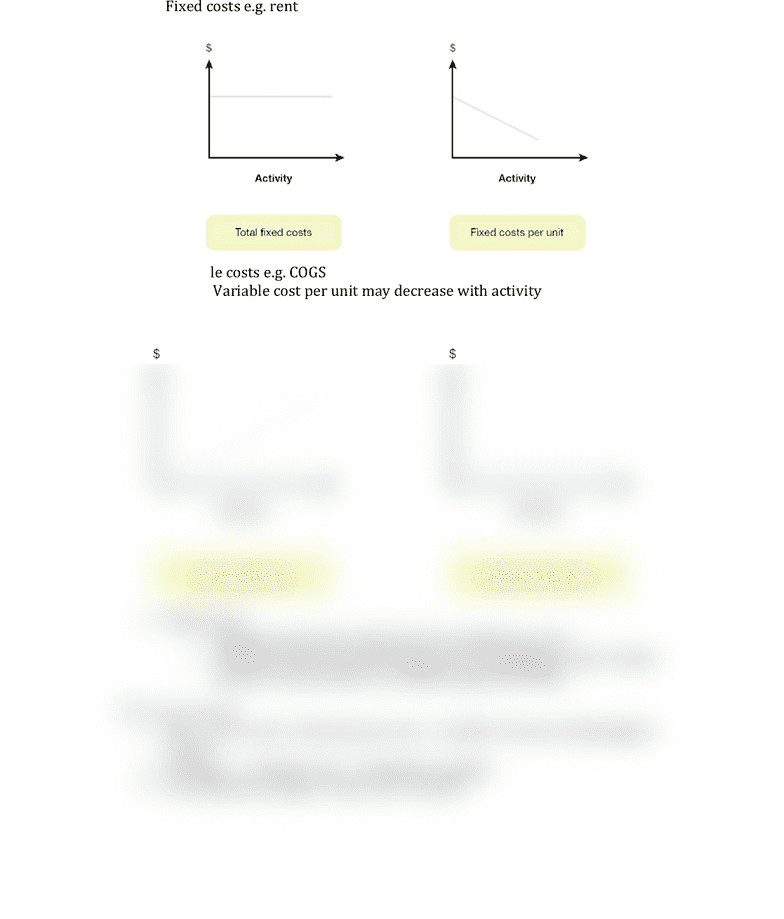Get 2 days of unlimited access
Class Notes (1,000,000)
AUS (30,000)
ANU (1,000)
Sorin (10)
Lecture 11

# BUSN1001 Lecture Notes - Lecture 11: Variable Cost

Department
Course Code
BUSN1001
Professor
Sorin
Lecture
11

This preview shows half of the first page. to view the full 2 pages of the document.Lecture 11
CVP (cost-profit analysis)
Fixed costs e.g. rent
Variable costs e.g. COGS
o Variable cost per unit may decrease with activity
Mixed costs
o Stepped costs (same until a level is reached) e.g. rent
o Semi fixed costs e.g. advertising costs designing a video is fixed,
amount of times shown in variable (aspects of both)
Break even point
BEP = Fixed costs / (Revenue per unit Variable costs per unit(marginal
costs))
Revenues variable costs = contribution margin
If contribution margin > fixed costs profit
###### You're Reading a Preview

Unlock to view full version